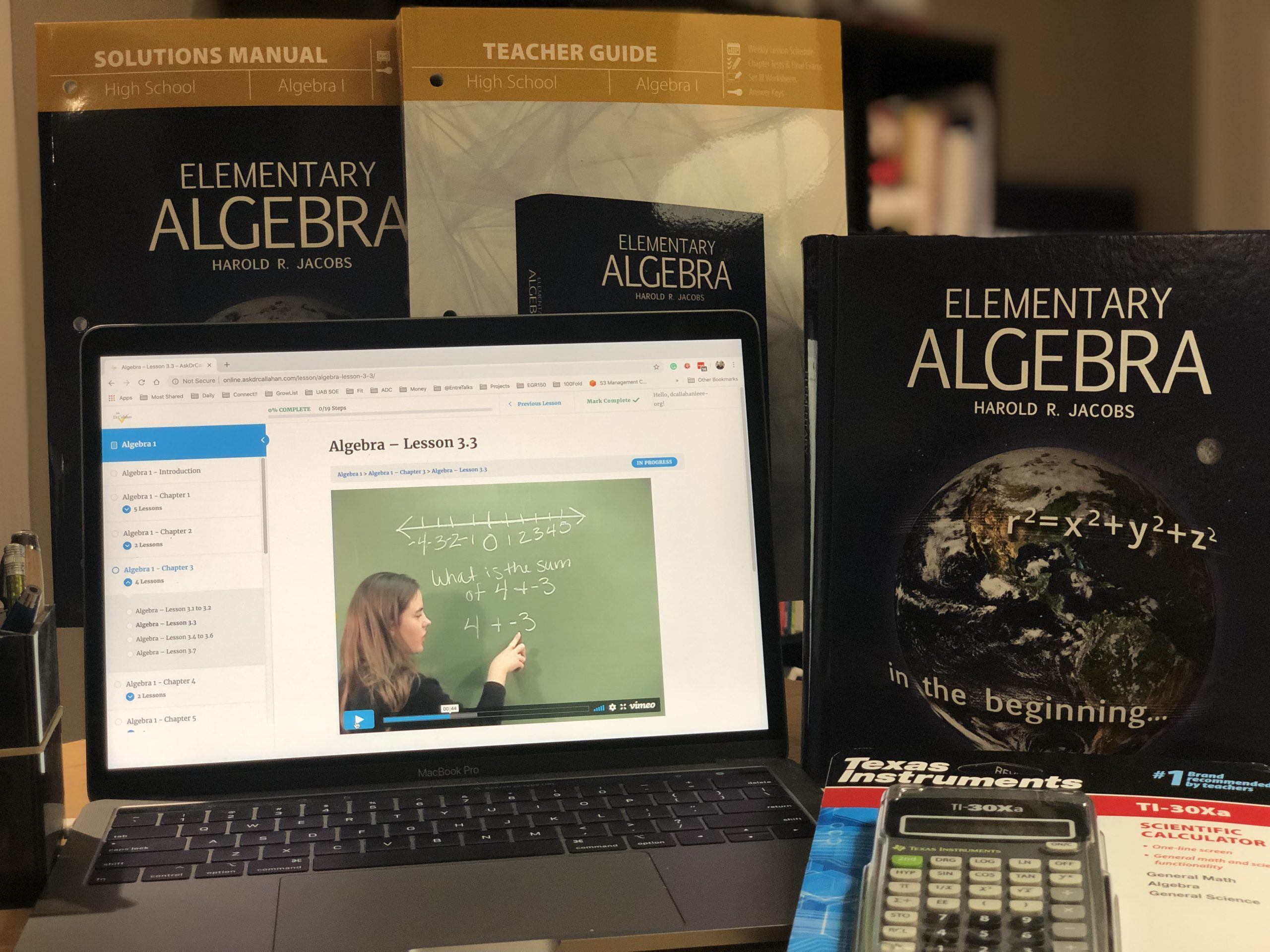Posted on

# Algebra Course Description for TranscriptIf you are looking for details for a transcript for Elementary Algebra by Jacobs course, here is the description you need.

Elementary Algebra (this course is the equivalent of an Algebra I course) provides a formal development of the algebraic skills and concepts necessary for students to succeed in advanced courses such as Geometry, Algebra II, Trigonometry, and then Calculus. Students who complete Algebra 1 should take Geometry next. Concepts from this course show up on the ACT and the SAT.

Topics Covered in this Course:

• Fundamental Operations
• Functions and Graphs
• Integers
• Rational Numbers
• Equations in One Variable
• Equations in Two Variables
• Simultaneous Equations
• Exponents
• Polynomials
• Factoring
• Fractions
• Square Roots